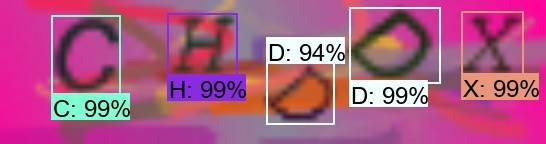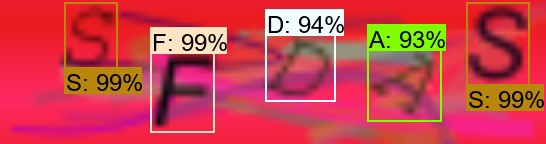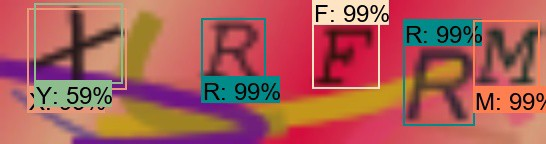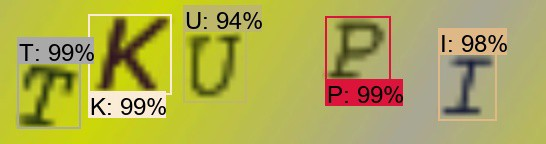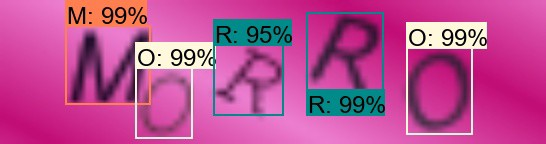# TensorFlow验证码解算器检测教程

C:\TensorFlow\research\object_detection>tensorboard --logdir=CAPTCHA_training_dirpython export_inference_graph.py --input_type image_tensor --pipeline_config_path CAPTCHA_training/faster_rcnn_inception_v2_coco.config --trained_checkpoint_prefix CAPTCHA_training_dir/model.ckpt-XXXX --output_directory CAPTCHA_inference_graph

# Welcome to the object detection tutorial !# Importsimport cv2import numpy as npimport osimport sys# run on CPUos.environ['CUDA_VISIBLE_DEVICES'] = '-1'import tensorflow as tffrom distutils.version import StrictVersionfrom collections import defaultdict# title of our windowtitle = "CAPTCHA"# Env setupfrom object_detection.utils import ops as utils_opsfrom object_detection.utils import label_map_utilfrom object_detection.utils import visualization_utils as vis_util# Model preparation PATH_TO_FROZEN_GRAPH = 'CAPTCHA_frozen_inference_graph_v2.pb'# List of the strings that is used to add correct label for each box.PATH_TO_LABELS = 'CAPTCHA_labelmap.pbtxt'NUM_CLASSES = 37# Load a (frozen) Tensorflow model into memory.label_map = label_map_util.load_labelmap(PATH_TO_LABELS)categories = label_map_util.convert_label_map_to_categories(label_map, max_num_classes=NUM_CLASSES, use_display_name=True)category_index = label_map_util.create_category_index(categories)detection_graph = tf.Graph()with detection_graph.as_default():    od_graph_def = tf.GraphDef()    with tf.gfile.GFile(PATH_TO_FROZEN_GRAPH, 'rb') as fid:        serialized_graph = fid.read()        od_graph_def.ParseFromString(serialized_graph)        tf.import_graph_def(od_graph_def, name='')# Detectiondef Captcha_detection(image):    with detection_graph.as_default():        with tf.Session(graph=detection_graph) as sess:            # Open image            image_np = cv2.imread(image)            # To get real color we do this:            image_np = cv2.cvtColor(image_np, cv2.COLOR_BGR2RGB)            # Expand dimensions since the model expects images to have shape: [1, None, None, 3]            image_np_expanded = np.expand_dims(image_np, axis=0)            # Actual detection.            image_tensor = detection_graph.get_tensor_by_name('image_tensor:0')            boxes = detection_graph.get_tensor_by_name('detection_boxes:0')            scores = detection_graph.get_tensor_by_name('detection_scores:0')            classes = detection_graph.get_tensor_by_name('detection_classes:0')            num_detections = detection_graph.get_tensor_by_name('num_detections:0')            # Visualization of the results of a detection.            (boxes, scores, classes, num_detections) = sess.run(              [boxes, scores, classes, num_detections],              feed_dict={image_tensor: image_np_expanded})            vis_util.visualize_boxes_and_labels_on_image_array(              image_np,              np.squeeze(boxes),              np.squeeze(classes).astype(np.int32),              np.squeeze(scores),              category_index,              use_normalized_coordinates=True,              line_thickness=2)            # Show image with detection            cv2.imshow(title, cv2.cvtColor(image_np, cv2.COLOR_BGR2RGB))            #cv2.imwrite("Predicted_captcha.jpg", cv2.cvtColor(image_np, cv2.COLOR_BGR2RGB))        Captcha_detection("11.jpg")